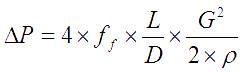# Reducer K value

## What is K factor of K value for piping fittings?

The frictional pressure drop across a length of a pipe depends on type of fluid, its density, viscosity etc, the inner surface of the pipe and fluid flow rate.

It is calculated using Darcy's equation.This equation represents the frictional pressure drop for the straight length of a pipe. But when different piping fittings and valves are also included in the pipe run, they modify the flow itself and that also contributes to the overall pressure loss.

K factor or K value for different piping fittings accounts for the additional frictional losses contributed by these fittings and valves. K value is then used to calculate the 'equivalent length' of fittings.

## Using K value to calculate frictional losses in a piping system

Equivalent length, Leq = K × (D/4f)

where, K is the K value for a fitting (or for all fittings combined)
D is the pipe diameter
f is the Fanning friction factor

You can use this equivalent length is used in the above Darcy's equation, to calculate the frictional pressure losses across the pipe run including all the fittings and valves in the path.

Note that the equivalent length calculated from above equation is added to the actual length of the pipe + fittings i.e. equivalent length represents the additional pressure loss caused by the shape of the fittings.

If the K factor is directly multiplied by (ρv2/g), that directly gives the additional pressure drop across corresponding fittings.

## K value for piping reducer / expander

#### K factor calculator

For quick calculation of equivalent length and frictional losses across a pipe run, approximate K value for reducers and expander joints can be considered to be 0.5.

You can also use this K factor calculator for different piping fittings for a quick estimation of the equivalent length.

#### Manually calculate K factor for reducer (or expander)

Alternatively, you can also use the following equations to calculate the K factor for reducer / expander joints.

K value for sudden contraction